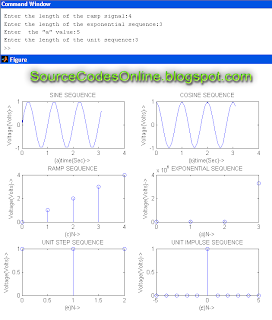# MATLAB Program for the generation of signals like Sine sequence, Cosine sequence, Ramp sequence, Exponential sequence, Unit-step sequence and Unit-impulse sequence | IT1254 - DSP and Communications Systems Lab

AIM:
To write a program using MATLAB for the generation of signals like Sine sequence, Cosine sequence, Ramp sequence, Exponential sequence, Unit-step sequence and Unit-impulse sequence in IT1254 - DSP and Communications Systems Lab.

ALGORITHM:

SINE SEQUENCE:
Step 1: Initialize the time period.
Step 2: Design the function of sine wave.
Step 3: Plot the sine sequence.
Step 4: Display the output

COSINE SEQUENCE:
Step 1: Initialize the time period.
Step 2: Design the function of cosine wave.
Step 3: Plot the cosine sequence.
Step 4: Display the output

RAMP SEQUENCE:
Step 1: Read the length of the ramp sequence.
Step 2: Define the length of the ramp sequence.
Step 3: Plot the ramp sequence using stem function.
Step 4: Display the output.

EXPONENTIAL SEQUENCE:
Step 1: Read the length of the exponential sequence.
Step 3: Plot the exponential sequence using stem function.
Step 4: Display the output.

UNIT-STEP SEQUENCE:
Step 1: Read the length of the unit-step sequence.
Step 2: Plot the unit-step sequence.
Step 3: Display the output.

UNIT-IMPULSE SEQUENCE:
Step 1: Read the length of the unit-impulse sequence.
Step 2: Plot the unit-impulse sequence.
Step 3: Display the output.

SOURCE CODE:
clc;
t=0:0.1:pi;
y=sin(2*pi*t);
subplot(3,2,1);
plot(t,y);
ylabel('Voltage(Volts)->');
xlabel('(a)time(Sec)->');
title('SINE SEQUENCE');
clc;
t=0:0.1:pi;
y=cos(2*pi*t);
subplot(3,2,2);
plot(t,y);
ylabel('Voltage(Volts)->');
xlabel('(b)time(Sec)->');
title('COSINE SEQUENCE');
clc;
n1=input('Enter the length of the ramp signal:');
t=0:n1;
subplot(3,2,3);
stem(t,t);
ylabel('Voltage(Volts)->');
xlabel('(c)N->');
title('RAMP SEQUENCE');
clc;
n2=input('Enter the length of the exponential sequence:');
t=0:n2;
a=input('Enter  the "a" value:');
y2=exp(a*t);
subplot(3,2,4);
stem(t,y2);
ylabel('Voltage(Volts)->');
xlabel('(d)N->');
title('EXPONENTIAL SEQUENCE');
clc;
n3=input('Enter the length of the unit sequence:');
t=0:1:n3-1;
y1=ones(1,n3);
subplot(3,2,5);
stem(t,y1);
ylabel('Voltage(Volts)->');
xlabel('(e)N->');
title('UNIT STEP SEQUENCE');
clc;
t=-5:5;
y=[zeros(1,5),ones(1,1),zeros(1,5)];
subplot(3,2,6);
stem(t,y);
ylabel('Voltage(Volts)->');
xlabel('(f)N->');
title('UNIT IMPULSE SEQUENCE');
OUTPUT:Click to view full image!

RESULT:
Thus the generation of signals was performed using MATLAB and the waveforms were plotted.

Previous
Next Post »